Introduction to Scatterplots

A scatterplot displays a relationship between two sets of data. A scatterplot can also be called a scattergram or a scatter diagram.

In a scatterplot, a dot represents a single data point. With several data points graphed, a visual distribution of the data can be seen.

Depending on how tightly the points cluster together, you may be able to discern a clear trend in the data.

The closer the data points come to forming a straight line when plotted, the higher the correlation between the two variables, or the stronger the relationship.

If the data points make a straight line going from near the origin out to high y-values, the variables are said to have a positive correlation. If the data points start at high y-values on the y-axis and progress down to low values, the variables have a negative correlation.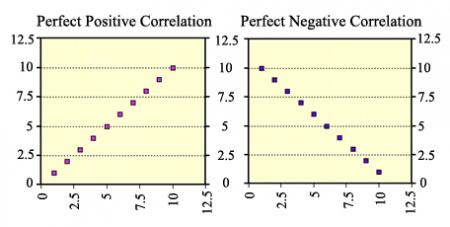An example of a situation where you might find a perfect positive correlation, as in the graph on the left above, would be if you were purchasing candy bars for \$1 each. As number of candy bars increase, the amount of total cost increases.

Hint: If you read the graph like you read a book from left to right, you can read the trend as increasing (positive) or decreasing (negative).

A situation where you might find a strong (but not perfect) positive correlation would be if you examined the number of hours students spent studying for an exam vs. the grade received. This won't be a perfect correlation because two people could spend the same amount of time studying and get different grades. But in general, the rule will hold true that as the amount of time studying increases so does the grade received.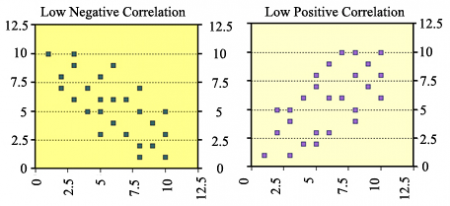Notice that the data points are spread out even more in these graphs. The closer the data points lie together to make a line, the higher the correlation.

In these graphs, there is still a trend in the data, so we would say that the data has a weak or lower correlation.

Take a look at the following graph. What do you notice?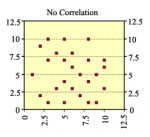The data points are spread out even more in this graph. This means there is no trend to the data; thus, there is no correlation.

Examples 1 to 3 of Real-Life Correlations

Let’s look at some real examples of data correlation.

Example 1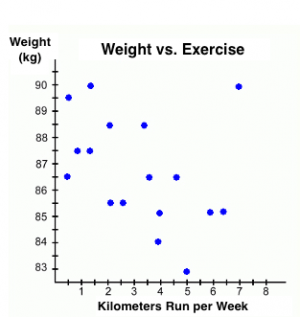This graph illustrates how a person's weight might change depending on how much they run in a week. It records the change in weight for a group of people, all of whom started out weighing 90kg. Each person runs a different number of kilometers each week for an unspecified period of time.

You can conclude from the graph that as the number of kilometers run each week increases, a person's weight decreases.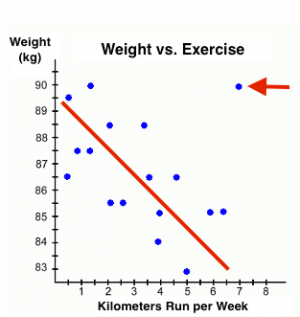When points are graphed on a scatterplot, it is possible to find a line of best fit—a straight line that best represents the data on a scatterplot. Here's the same graph with the line of best fit drawn in. Notice again that the points only "sort of" line up. That's why it's a weak negative correlation.

But notice also the point in the upper right of the graph (red arrow). This data element is an anomaly. It doesn't fit the pattern of the other points and we didn't use it when drawing the line of best fit. We call that an outlier—a straight line that best represents the data on a scatterplot.

But we still have to explain it. Why is it there?

This outlier point represents one person who ran 7 km every week, but whose weight stayed at 90 kg. We might search for an explanation, perhaps even interviewing that person, and discover that the only food that person ever eats is fatty fast food . . . thus explaining his or her lack of weight loss!

Example 2

Emily kept a record of the number of hours she studied and the test grades that she received. Examine the graph of this relationship and determine if it shows a positive correlation, a negative correlation, or no correlation. If there is a positive or negative correlation, describe its meaning in the situation.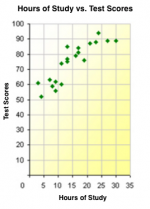Example 3

This graph shows how a chemical reacts to changing temperature. Determine whether the graph shows a positive correlation, a negative correlation, or no correlation. If there is a positive or negative correlation, describe its meaning in the situation.

Examples 4 to 6 of Real-Life Correlations

Example 4

Which graph is the best example of a negative correlation? (Click on each image to check your answer.)

Example 5

This graph shows the height and arm span for a group of 10 people.  Determine whether the graph shows a positive correlation, a negative correlation, or no correlation. If there is a positive or negative correlation, describe its meaning in the situation.

Example 6

This graph shows the age of 20 people and the number of pets they own. Determine whether the graph shows a positive correlation, a negative correlation, or no correlation. If there is a positive or negative correlation, describe its meaning in the situation.